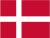### Dish Cloth 13

Cast on  60 stitches and knit 6 rows . Then knit the pattern but remember to begin  every row with 2 k and end every row with 2 k.

Pattern:

1.row: knit
2.row: purl
3. - 5. - 7.row: 2 k, 1 p, 1 k, 1 p, 1 k, 1 p, 2 k, 5 p
4. - 6. - 8.row: 5 k, 3 p, 1 k, 1 p, 1 k, 3 p
9.row: knit
10. row: purl
11. - 13. - 15.row: 2 k, 5 p, 2 k, 1 p, 1 k, 1 p, 1 k, 1 p
12. - 14. - 16.row: 1 p, 1 k, 1 p, 1 k, 3 p, 5 k, 2 p

 X X X X X X X 16 X X X X X X X X 15 X X X X X X X 14 X X X X X X X X 13 X X X X X X X 12 X X X X X X X X 11 10 9 X X X X X X X 8 X X X X X X X X 7 X X X X X X X 6 X X X X X X X X 5 X X X x X X X 4 X X X X X X X X 3 2 1

There are a lot of patterns on Pinterest. I made some mistakes in the beginning when reading the patterns. You have to remember that all uneven rows start in the right side of the diagram and X=purl and ▢= knit. All equal rows start in the  left side of the diagram and ▢= purl and X=knitDanish